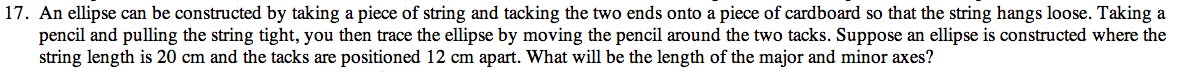13E-17.

### Missing problem for Book PCT => Chapter Ch13 => Lesson 13.1.2 => Problem 13E-17

Created from orphaned homework help problemIf the string length is 20 cm and the remaining length is evenly divided into two segments, what would one segment's length be? This length would be the hypotenuse of the right triangle from one foci and the center.

The length would be 4 cm.